Select Page

# Fuzzy AHP (chang) steps

Step1.The fuzzy synthetic extent value (Si) with respect to the ith criterion is defined as equation 1 .

To obtain equation 2;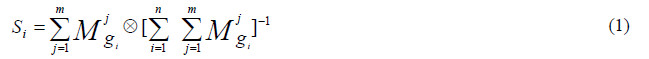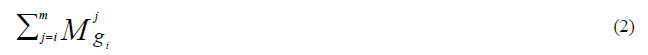perform the “fuzzy addition operation” of m extent analysis values for a particular matrix given in equation 3 below, at the end step of calculation, new (l, m, u) set is obtained and used for the next: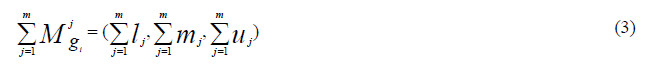Where l is the lower limit value, m is the most promising value and u is the upper limit value.
and to obtain equation 4;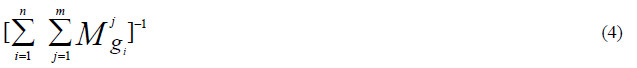perform the “fuzzy addition operation” of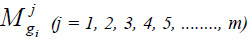values give as equation 5: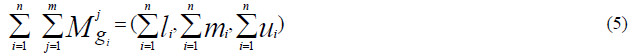and then compute the inverse of the vector in the equation (5) equation (6) is then obtained such that

Step 2. The degree of possibility of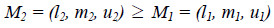is defined as equation 7: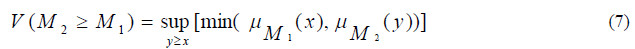and x and y are the values on the axis of membership function of each criterion. This expression can be equivalently written as given in equation 8 below: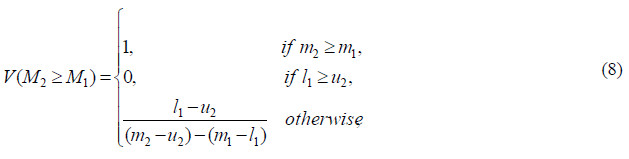where d is the highest intersection point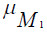and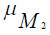(see Figure 1)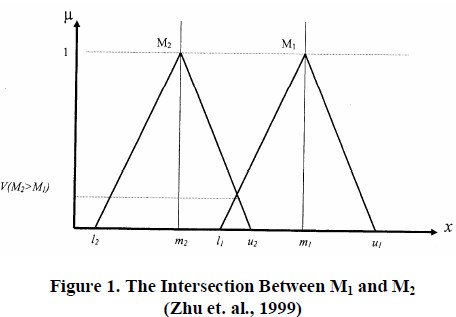To compare M1 and M2; we need both the values of V(M2≥ M1) and V(M1≥M2):

Step 3. The degree possibility for a convex fuzzy number to be greater than k convex fuzzy numbers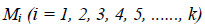can be defined by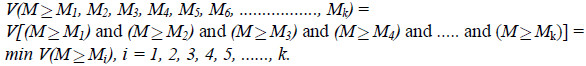Assume that equation 9 is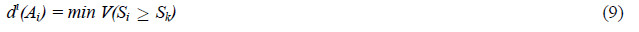For k = 1, 2, 3, 4, 5, ……, n; k ≠ i. Then the weight vector is given by equation 10: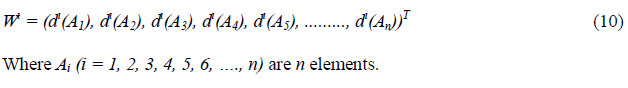Step 4. Via normalization, the normalized weight vectors are given in equation 11: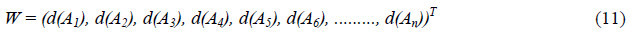Where W is non-fuzzy numbers.